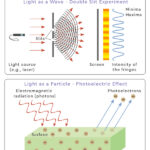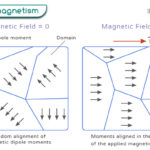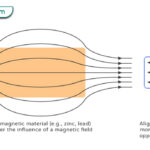Home / Physics / Simple Machines

# Simple Machines

## What are Simple Machines

Simple machines are mechanical devices that use a force to do valuable work by changing the direction or magnitude of the force. They have a simple mechanism accompanied by no or very little motion. For example, a pair of scissors is a simple machine that can easily cut through paper.

Greek philosopher Archimedes was the first to study and quantify simple machines in the 3rd century B.C.

## How do Simple Machines Work

The basic principle of simple machines is that a force is applied at one point and transmitted to another point. The applied force is the input force manifested at the output as the output force.

Simple machines can accomplish one or more of the following functions.

• Transfer force from one point to another
• Change the direction of the force
• Increase the magnitude of the force
• Increase the distance or speed of the force

A torque is necessary for the machine to function whenever a rotational motion is involved. Torque is defined as the product of the force and the distance about which rotation takes place.

## Mechanical Advantage of Simple Machines

Simple machines work by providing leverage, known as mechanical advantage. They multiply the input force, making it easier to use them. The ideal mechanical advantage (IMA) of a simple machine is mathematically defined as the ratio of the output force and input force.

Ideal Mechanical Advantage = Output force/ Input force

The equation shows that the higher the mechanical advantage, the lower is the input force.

Because machines are not perfect, there will be some energy loss due to friction. Hence, the mechanical advantage obtained from simple machines is the actual mechanical advantage (AMA). The actual to ideal mechanical advantage ratio gives the machine efficiency.

Efficiency = AMA/IMA x 100%

In other words, no machine can be 100% efficient.

## Types of Simple Machines

There are six types of simple machines. Most of them are used in everyday life and are commonly found in homes.

### 1. Lever

A lever consists of a beam resting on a support. When a force is applied at a point on the beam, it rotates about the support point. The function of a lever is to multiply the applied force so that a heavy object can be raised. The applied force is called effort, the object is the load, and the support is the fulcrum or pivot. Depending on the position of the effort, fulcrum, and load, a lever can be of three types – first, second, and third class. A lever provides a higher mechanical advantage when the distance between the fulcrum and effort is longer.

Examples: Seesaw, crowbar, scissors, and wheelbarrow

### 2. Inclined Plane

An inclined plane is the simplest of all simple machines. It does not consist of any moving parts, neither does it move. An inclined plane consists of a slope that makes a fixed angle to the surface on which it rests. It moves an object from a lower level to a higher level without taking it vertically. In other words, the force applied to the object is less than its weight. A more extended inclined plane provides a higher mechanical advantage, and the object can be raised will less effort.

Examples: Ramp, slide, sloping road, and funnel

### 3. Wedge

A wedge has a triangular shape and comprises one or two incline planes. A wedge made up of two incline planes has a sharp edge when the planes meet. It is mainly used to slice, cut, split, and lift an object. A long and sharp wedge gives a better mechanical advantage than a short and blunt one. A wedge works by changing the direction of the applied force, enabling it to cut through materials. The difference between an inclined plane and a wedge is that a wedge moves, whereas an inclined plane remains still.

Examples: Knife, axe, scalpel, and shovel

### 4. Wheel and Axle

A wheel and axle consists of a large disc (wheel) and a long rod or shaft (axle). A rotational force or torque is applied to one part, which then rotates and transmits the force to the other part. Mechanical advantage is gained by increasing the size of the wheel. A wheel and axle has many functions, including transporting and lifting objects. Bicycles and cars are equipped with wheels and axles to roll over large distances. Besides, rolling over a surface generates less friction than sliding.

Examples: Screwdriver, potter’s wheel, merry-go-round, and windmill

### 5. Pulley

A pulley consists of a wheel that rotates about its axis. A rope passing over the wheel is pulled to lift objects. Combining two or more pulleys allows one to raise heavy objects off the ground using minimum effort. In this way, the mechanical advantage is increased. Based on its design, a pulley can be of three types – fixed pulley, moveable pulley, and compound pulley.

Examples: Pulleys can be found in a flagpole, construction crane, water well, and elevator.

### 6. Screw

A screw is a circular device in which a narrow inclined planed is wrapped around a cylinder, like a spiral staircase. One end of the screw forms the head, and the other end is sometimes pointed and forms the tip. A screw works on the principle of converting circular motion into translational motion. When a rotational force or torque is applied to the head, the screw rotates and moves linearly. A screw consists of threads, and the distance between each thread is called the pitch. The mechanical advantage can be gained by increasing the pitch. A screw is used for holding things, drilling holes, and lifting objects.

Examples: Bottle cap, corkscrew, car jack, and light bulb

The following image summarizes all the simple machines.

The following image shows examples of simple machines in everyday life.Wave-Particle DualityPhotoelectric EffectFerromagnetismDiamagnetism